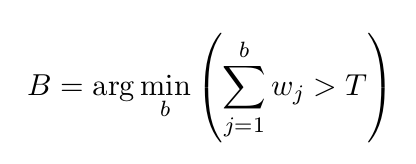# Department of Mathematics and Computer Science

www.univ-soukahras.dz/en/dept/mi

Module: Initiation à la recherche

1. Information
2. Questions

## Writing Equations using Latex (2)

 2 votes What's the latex code for the following Equation:Asked on 19:27, Monday 16 May 2016 By Imed BOUCHRIKA In Initiation à la recherche

 2 votes \begin{equation} B = \arg \min_{n}(\sum_{j=1}^n \omega_{j} > T) \end{equation} Answered on 18:55, Tuesday 17 May 2016 by Hanen Rouainia(322 points) In Initiation à la recherche
 1 votes \begin{equation} B = arg ~ \underset{b} min ( \sum_{\substack{j = 1}}^{b} w_j > T)\end{equation} Answered on 20:17, Friday 27 May 2016 by amirat abdallah(257 points) In Initiation à la recherche